Latest Banking jobs   »

# Quantitative Aptitude Quiz For IBPS RRB PO/Clerk Mains 2022- 3rd September

Directions (1-5): Bar chart given below shows markup % and discount % on five different articles sold by a shopkeeper. Study the data carefully and answer the following questions.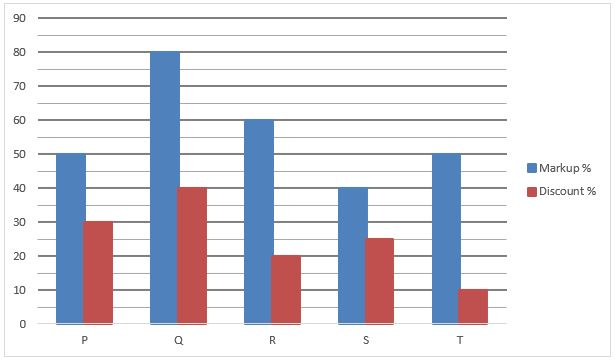Q1. If ratio of selling price of article ‘P’ to that of article ‘T’ is 1 : 1, then find out the ratio of cost price of article ‘P’ to that of article ‘T’?
(a) 9 : 5
(b) 9 : 11
(c) 7 : 9
(d) 9 : 7
(e) 9 : 4

Q2. Cost price of article ‘Q’ is Rs.100 more than Cost price of article ‘R’ while mark price of article ‘Q’ is Rs.260 more than mark price of article ‘R’. Find the difference between selling price of article ‘Q’ and that of article ‘R’?
(a) Rs. 28
(b) Rs. 24
(c) Rs. 36
(d) Rs. 18
(e) Rs. 48

Q3. If shopkeeper earn Rs.48 profit on selling article ‘S’, then find the Mark price of article ‘S’?
(a) Rs. 1348
(b) Rs. 1344
(c) Rs. 1364
(d) Rs. 1324
(e) Rs. 1304

Q4. Selling price of article ‘Q’ is 68.75% more than that of article ‘R’, then cost price of article ‘Q’ is what percent more than that of article ‘R?
(a) 125%
(b) 75%
(c) 150%
(d) 112.5%
(e) 100%

Q5. Which article is sold at second maximum profit if cost price of each article is same?
(a) P
(b) S
(c) T
(d) R
(e) Q

Directions (6 – 10): What will come in the place of question (?) mark in the following number series:

Q6. 12, 7, 8.5, 14.75, ?, 83
(a) 30
(b) 24
(c) 32
(d) 36
(e) 48

Q7. 12, 78, 395, 1584, 4755, ?
(a) 9512
(b) 9516
(c) 9518
(d) 9520
(e) 9580

Q8. 26, 53, 214, 1287, 10300, ?
(a) 95000
(b) 100005
(c) 103000
(d) 101005
(e) 103005

Q9. 4187, 2857, 2129, 1787, 1663, ?
(a) 1647
(b) 1642
(c) 1627
(d) 1637
(e) 1630

Q10. 27, 27, 54, 18, 72, ?
(a) 19.6
(b) 16.8
(c) 18.8
(d) 12.8
(e) 14.4

Q11. If curved surface area and total surface area of a cylinder are 330m² and 1023m² respectively. Find ratio of radius to height of cylinder?
(a) 10 : 7
(b) 7 : 5
(c) 21 : 10
(d) 21 : 5
(e) Can’t be determined

Q12. A started a business with Rs 50000 and after some month B joined the business with Rs 60000. Again, after some month C joined the business with Rs 75000. At the end of the year C got Rs. 9150 out of total profit of Rs 33550 and profit share of A and B is equal. Find how many months after B joined the business did C join?
(a) 4 months
(b) 6 months
(c) 10 months
(d) 3 months
(e) none of these.

Q13. Two person of equal ability starts making a wall. First person works for x hours for x day and second person works for y hours for y days and they complete the wall. If first person works alone for it’s respective hours per day then it takes 33⅓ day to complete the wall and if second person works alone for it’s respective hours then it takes 25 days to complete the wall. Find (y–x)?
(a) 3
(b) 4
(c) 2
(d) 5
(e) 1

Q14. Sum of present age of A and C is 75 years. Ratio of age of A five years ago to age of B five years hence is 5:8, and ratio of age of B five years ago to present age of C is 2:3. Find difference between present age of A and B?
(a) 10 years
(b) 5 years
(c) 3 years
(d) 8 year
(e) 6 year

Q15. B is 40% less efficient than A who can complete the work in 50% less time than C. If 75% of total works can be completed in 15 day by B working alone then find in how many day A and C working together can complete the 80% of the work?
(a) 6.4 days
(b) 4 days
(c) None of these
(d) 4.6 days
(e) 6⅔ days

Solutions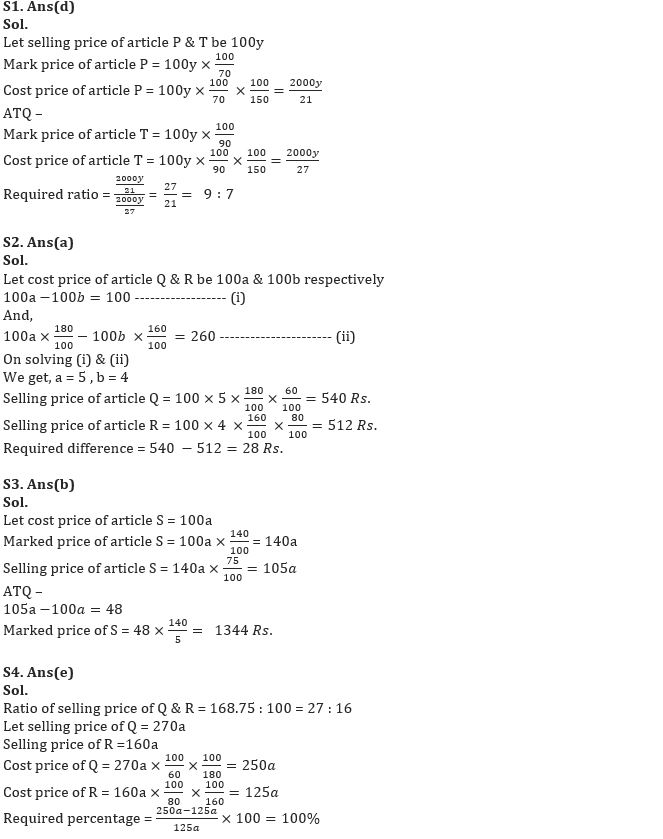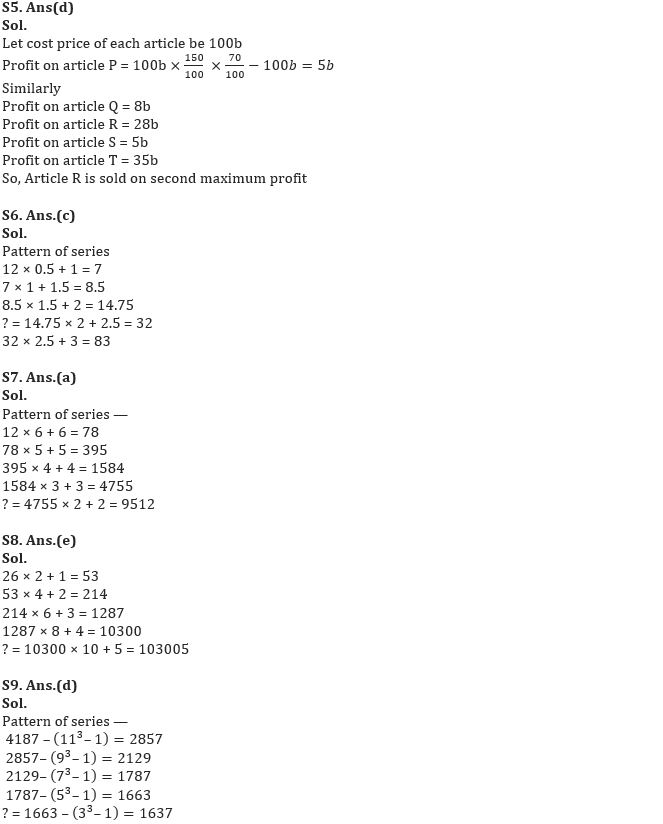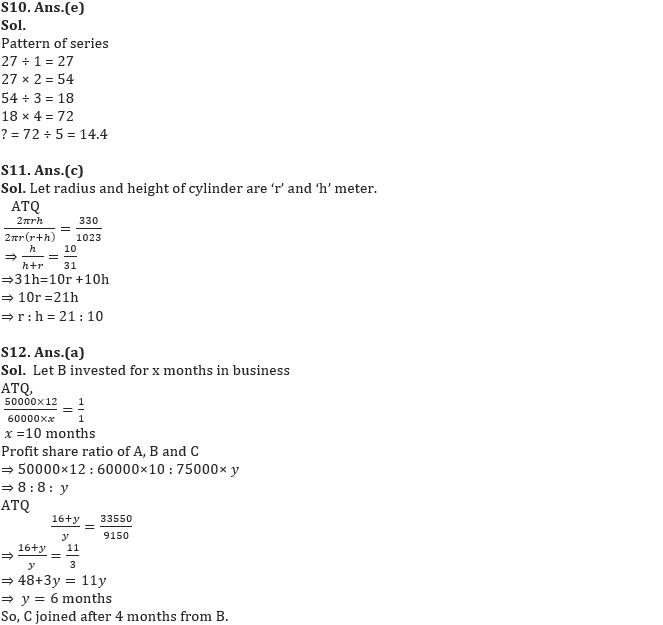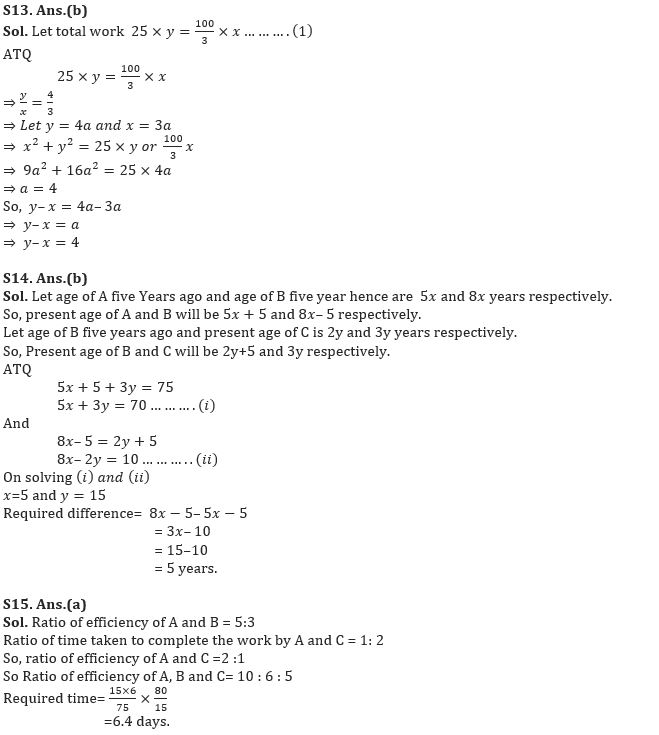#### Congratulations!Download Hindu Review of October 2021: Free PDF